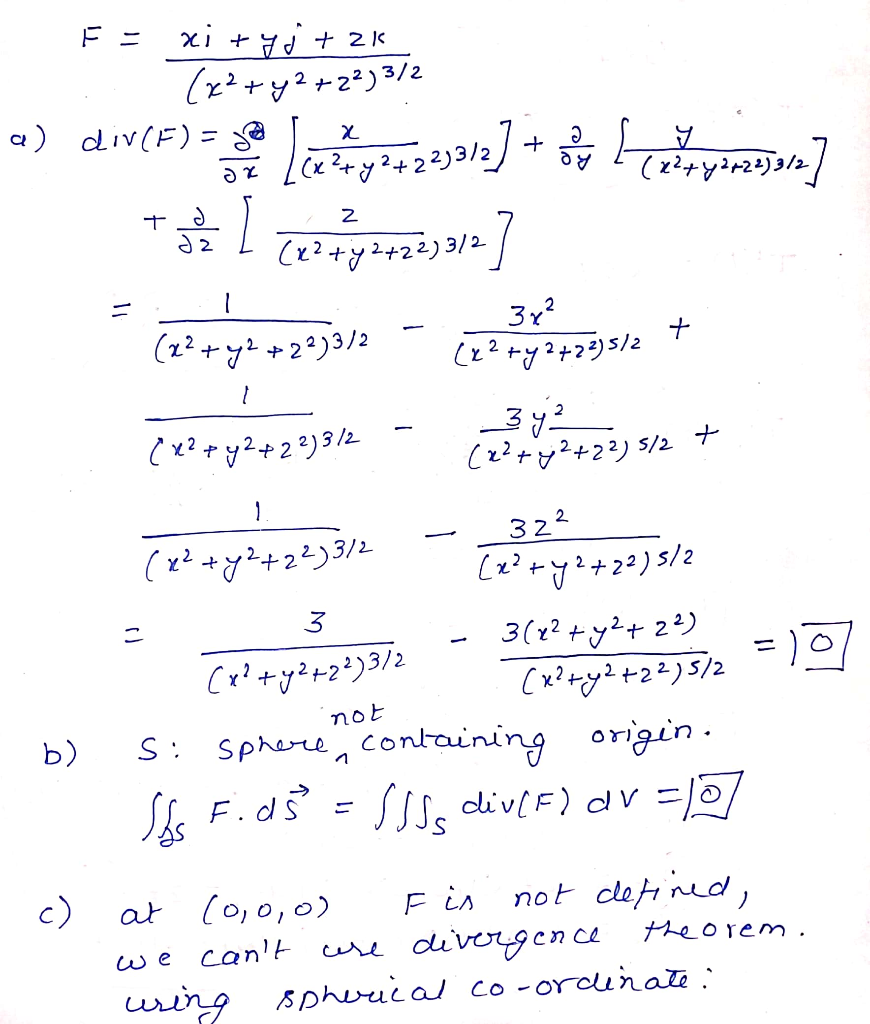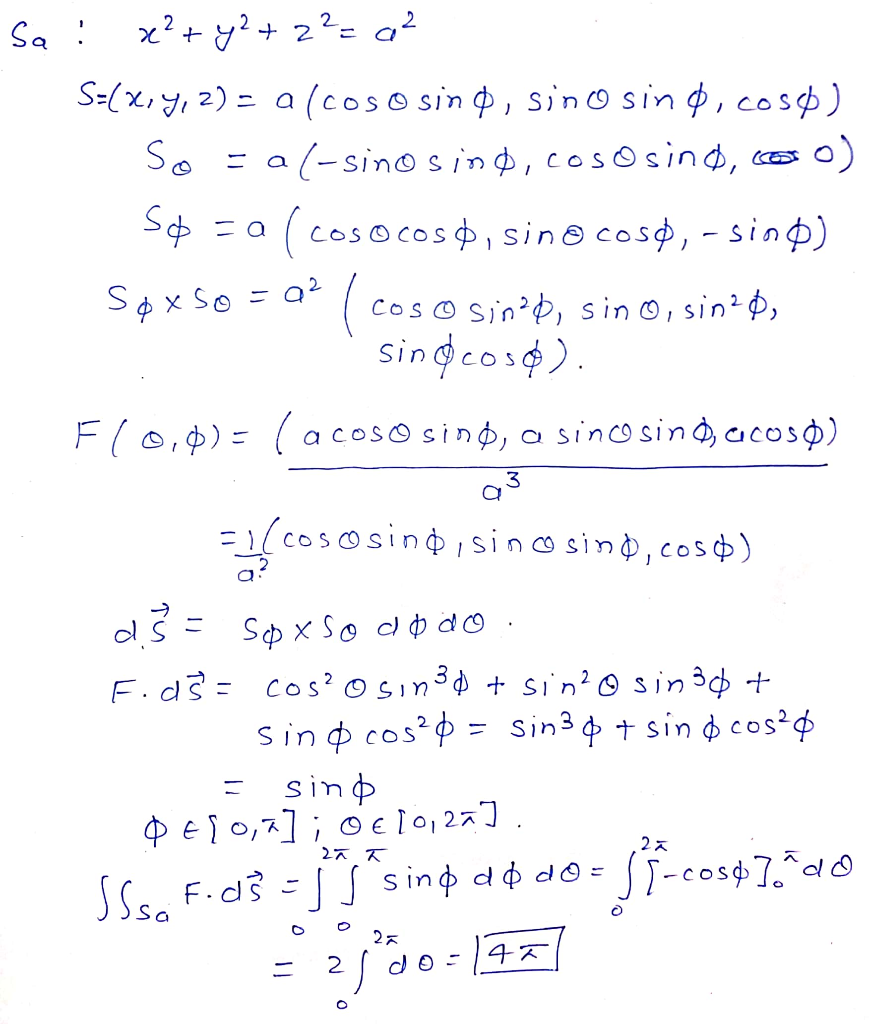# Consider the following vector ﬁeld. F = (xi + yj + zk )/((x^2 + y^2 + z^2)^3/2) (a) Find the divergence of F. (b) Let S...

Consider the following vector ﬁeld. F = (xi + yj + zk )/((x^2 + y^2 + z^2)^3/2)

(a) Find the divergence of F.

(b) Let S be any sphere not containing the origin. Find the outward ﬂux of F across S.

(c) Let Sa be the sphere of radius a centered at the origin. Find the outward ﬂux of F across Sa.##### Add Answer of: Consider the following vector ﬁeld. F = (xi + yj + zk )/((x^2 + y^2 + z^2)^3/2) (a) Find the divergence of F. (b) Let S...
More Homework Help Questions Additional questions in this topic.